# Voltage: What is it? (Definition, Formula And How To Measure Potential Difference)

Contents

## What is Voltage?

Voltage (also known as electric potential difference, electromotive force emf, electric pressure, or electric tension) is defined as the electric potential difference per unit charge between two points in an electric field. Voltage is expressed mathematically (i.e. in formulas) using the symbol “V” or “E”.

If you’re looking for a more intuitive explanation to help explain what voltage is, skip to this section of the article.

Otherwise, we’ll continue below with a more formal definition of voltage.

In a static electric field, the work required to move per unit of charge between two points is known as voltage. Mathematically, the voltage can be expressed as,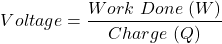Where work done is in joules and charge is in coulombs.We can define voltage as the amount of potential energy between two points in a circuit.

One point has a higher potential and the other points have lower potential. The difference in charge between higher potential and lower potential is called a voltage or potential difference.

The voltage or potential difference gives the force to the electrons to flow through the circuit.

The higher the voltage, the greater the force, and hence the more electrons flowing through the circuit. Without voltage or potential difference, electrons would move randomly in free space.

Voltage is also sometimes referred to as “electric tension”. For example, the voltage handling capacity of cables such as 1 kV, 11 kV, and 33 kV are referred to as low tension, high tension, and super tension cables respectively.

### Definition of Potential Difference as Potential of Electric Field

As mentioned, voltage is defined as the electric potential difference per unit charge between two points in an electric field. Let us describe this using equations.

Consider two points A and B.

The potential of point A with respect to point B is defined as the work done in moving a per unit charge from point A to B in the presence of electric field E.

Mathematically, this can be expressed as,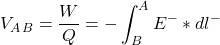This is also a potential difference between points A and B with point B as a reference point. It can also be expressed as,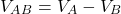Now voltage can be quite a difficult concept to understand conceptually.

So we’ll use an analogy to something tangible—something in the real world—to help make voltage easier to understand.

## Understanding Voltage By Analogy

The “Hydraulic analogy” is a common analogy used to help explain voltage.

In the hydraulic analogy:

• The voltage or electric potential is equivalent to hydraulic water pressure
• Electric current is equivalent to hydraulic water flow rate
• Electrical charge is equivalent to a quantity of water
• An electrical conductor is equivalent to a pipe

### Analogy 1

Consider a water tank as shown in the below figure. Figure (a) shows two tanks filled with the same water level. So, water can not flow from one tank to another tank as there is no pressure difference.

Now, Figure (b) shows two tanks filled with different water levels. Hence there is some pressure difference between these two tanks. Thus, water will flow from one tank to another tank till the water level of both tanks becomes equal.

Similarly, if we connect two batteries through conducting wire with different voltage levels then charges can flow from the battery of higher potential to the battery of lower potential. Hence, the battery of lower potential gets charged till the potential of both the battery becomes the same.

### Analogy 2

Consider a water tank placed at a certain height above the ground. There is a hose at the bottom of the tank as shown in the below image.

The water pressure at the end of the hose is equivalent to the voltage or potential difference in an electric circuit. The water in the tank is equivalent to the electric charge. Now if we increase the amount of water in the tank then more pressure is developed at the end of the hose.

Conversely, if we drain a certain amount of water from the tank then the pressure created at the end of the hose will decrease. We can assume this water tank is like a storage battery. When the voltage of the battery decreases the lamps get dimmer.

### Analogy 3

Let us understand how work can be done by voltage or potential difference in an electric circuit. A simple analogy between the hydraulic water circuit and the electric circuit is shown in the below figure.

As shown in the hydraulic water circuit, water flows through a pipe driven by a mechanical pump. A pipe is equivalent to conducting wire in an electric circuit.

Now, if a mechanical pump produces a pressure difference between two points, then pressurized water will be able to do work, such as driving a turbine.

Similarly, in an electric circuit, the potential difference of a battery can cause current to flow through the conductor hence, work can be done by flowing electric current, such as lighting the lamp.

## What is Voltage Measured In (Voltage Units)?

### SI Unit of Voltage

The SI unit for voltage is volts. This is represented by a V. Volt is a derived SI unit of voltage. The Italian physicist Alessandro Volta (1745-1827), who invented the voltaic pile, which was the first electrical battery hence, the unit volt is named in honor of them.

### Volt in SI Base Units

Volt can be defined as the electric potential difference between two points in an electric circuit that dissipates one joule of energy per coulomb of charge that passes through the electric circuit. Mathematically, it can be expressed as,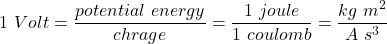Hence, volt can be expressed in terms of SI base units asor.

It can also be measured in watts per ampere or ampere times ohms.

## Voltage Formula

The basic formula for the voltage is shown in the below image.

### Voltage Formula 1 (Ohm’s Law)

According to Ohm’s law, voltage can be expressed as,#### Example 1

As shown in the below circuit a current of 4 A is flowing through the resistance of 15 Ω. Determine the voltage drop across the circuit.

Solution:

Given Data: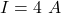,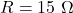According to Ohm’s law,Thus, by using the equation we get a voltage drop across the circuit of 60 volts.

### Voltage Formula 2 (Power And Current)

The power transferred is the product of supply voltage and electric current.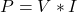Now, put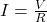in the above equation we get,

(1)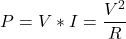Thus, we get voltage equals the power divided by current. Mathematically,#### Example 2

As shown in the below circuit a current of 2 A is flowing through a lamp of 48 W. Determine the supply voltage.

Solution:

Given Data: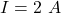,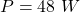According to the formula between voltage, power, and current mentioned above,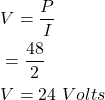Thus, by using the equation we get a supply voltage of 24 volts.

### Voltage Formula 3 (Power And Resistance)

According to equation (1), Voltage is the square root of the product of power and resistance. Mathematically,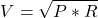#### Example 3

As shown in the below circuit determine the necessary voltage to glow a 5 W lamp with a resistance of current of 2 Ω.

Solution:

Given Data:,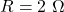According to the formula mentioned above,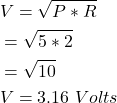Thus, by using the equation we get the necessary voltage to glow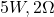lamp 3.16 Volts.

## Voltage Circuit Symbol (AC And DC)

### AC Voltage Symbol

The symbol for AC (alternating current) voltage is shown below:

### DC Voltage Symbol

The symbol for DC (direct current) voltage is shown below:

## Dimensions of Voltage

Voltage (V) is a representation of the electric potential energy per unit charge.

The dimensions of voltage can be expressed in terms of mass (M), length (L), time (T), and ampere (A) as given by.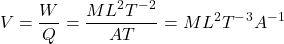Note that some also use I in place of A to represent current. In this case, the dimension of voltage can instead be represented as.

## How to Measure Voltage

In an electrical and electronic circuit, a voltage measurement is an essential parameter that needs to be measured. We can measure the voltage between a particular point and the ground or zero-volt line on a circuit.

In a 3-phase circuit, if we measure the voltage between any of one phase from the 3-phase and neutral point then it is known as line-to-ground voltage.

Similarly, if we measure the voltage between any two phases from 3-phase then it is known as line-to-line voltage.

There are various instruments used to measure the voltage. Let’s discuss each method.

### Voltmeter Method

The voltage between two points in a system can be measured by using a voltmeter. To measure a voltage, a voltmeter must be connected in parallel with the component whose voltage is to be measured.

One lead of the voltmeter must be connected to the first point and one to the second point. Note that the voltmeter should never be connected in series.

Voltmeter can also be used to measure the voltage drop across any component or the sum of the voltage drop across two or more components within a circuit. The below image shows the voltmeter connection for the measurement of voltage across the resistor.

An analog voltmeter works by measuring the current through a fixed resistor. Now, according to Ohm’s law, the current through the resistor is directly proportional to the voltage or potential difference across the fixed resistor.  Thus, we can determine the unknown voltage.

Another example of a voltmeter connection for the measurement of voltage across a 9 V battery is shown in the figure below:

### Multimeter Method

In present days, one of the most common methods to measure the voltage is by using a multimeter. The multimeter can be either analog or digital but digital multimeters are most commonly used because of higher accuracy and low cost.

The voltage or potential difference across any equipment can simply be measured by connecting probes of a multimeter across the two points where the voltage is to be measured. The measurement of battery voltage by using a multimeter is shown in the below image.

### Potentiometer Method

The potentiometer works on the principle of the null balance technique. It measures the voltage by comparison of an unknown voltage with the known reference voltage. The potentiometer circuit for the measurement of voltage is shown in the below figure.

Other instruments like an oscilloscope, an electrostatic voltmeter can also be used to measure the voltage.

## Difference Between Voltage and Current (Voltage vs Current)

The major difference between voltage and current is that voltage is the potential difference of electrical charges between two points in an electric field, whereas the current is the flow of electrical charges from one point to the other point in an electric field.

We can simply say that the voltage is the cause of the current to flow whereas the current is the effect of voltage.

The higher the voltage, the more the current to flow between two points. Note that if two points in a circuit are at the same potential then current cannot flow between those points. The magnitude of a voltage and current depends on each other (as per Ohm’s law).

Other differences between voltage and current are discussed in the table below.

## Difference Between Voltage and Potential Difference (Voltage vs Potential Difference)

There is not much difference between the voltage and the potential difference. But we can describe the difference between them in the following ways.

The voltage is the amount of energy required to move a unit charge between two points whereas the potential difference is the difference between the higher potential of one point and the lower potential of the other point.

Due to point charge:

The voltage is the potential obtained at some point considering the other reference point at infinity. Whereas potential difference is the difference in potential between two points at finite distances from charge. Mathematically they can be expressed as,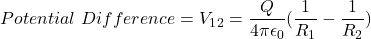If you would prefer a video explanation of voltage, check out the video below:

## What is a Common Voltage?

A common voltage is defined as the typical voltage level or rating of the electrical apparatus or equipment.

A list of common voltage for various electrical apparatus or equipment is listed below.

• Lead-acid batteries used in electric vehicles:  12 Volts DC. 12 V battery consists of 6 cells with a common voltage of each cell is 2.1 V. Note that cells are connected in series to increase voltage rating.
• Solar cells: Typically produce a voltage of around 0.5 Volts DC under open-circuit conditions. However, multiple solar cells are often connected in series to form solar panels, which can output a higher total voltage.
• USB: 5 Volts DC.
• High voltage electric power transmission line: 110 kV to 1200 kV AC.
• High-speed train (traction) power lines: 12 kV and 50 kV AC or 0.75 kV and 3 kV DC.
• TTL/CMOS power supply: 5 Volts.
• A single-cell, rechargeable nickel-cadmium battery: 1.2 Volts.
• Flashlight batteries: 1.5 Volts DC.

A common voltage supplied by the distribution company to residential consumers are:

• 100 V, 1-phase AC in Japan
• 120 V, 1-phase AC in America
• 230 V, 1-phase AC in India, Australia

A common voltage supplied by the distribution company to industrial consumers are:

• 200 V, 3-phase AC in Japan
• 480 V, 3-phase AC in America
• 415 V, 3-phase AC in India

## Applications of Voltage

Some of the applications of voltage include:

• One of the most common applications of the voltage is to determine the voltage drop across an electrical device or equipment such as a resistor.
• The addition of Voltage is required to increase the voltage rating. Hence, cells are connected in series to increase voltage rating.
• Voltage is the basic energy source of every piece of electrical and electronic equipment. From small voltages of (5 V) to high voltages (415 V) are used in various applications.
• Low voltage is usually used for many electronics equipment and control applications.
• High voltage is used for

Want To Learn Faster? 🎓
Get electrical articles delivered to your inbox every week.
No credit card required—it’s 100% free.